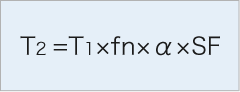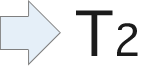##Calculate the joint capacity using the following formula, based on the figures obtained from(1)(2)(3) and (4) in.

■Calculation method of joint capacityT₂＝ joint capacity
T₁＝transmission torque
fn＝coefficient of revolution velocity
α＝coefficient of joint angle
SF＝safety factor*Note: Figures of maximum transmission torque capacity shown in each type and size are our company's own values under the premise that the joint angle is less than 10°and the service conditions of prime mover are considered.